Tamilnadu State Board New Syllabus Samacheer Kalvi 8th Maths Guide Pdf Chapter 1 Numbers Ex 1.3 Textbook Questions and Answers, Notes.

## Tamilnadu Samacheer Kalvi 8th Maths Solutions Chapter 1 Numbers Ex 1.3

Question 1.
Verify the closure property for addition and multiplication for the rational numbers $$\frac{-5}{7}$$ and $$\frac{8}{9}$$.
Let a = $$\frac{-5}{7}$$ and b = $$\frac{8}{9}$$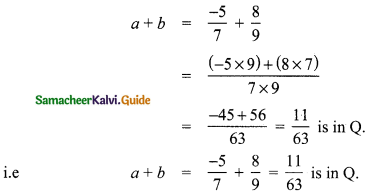∴ Closure property is true for addition of rational numbers.
Closure property for multiplication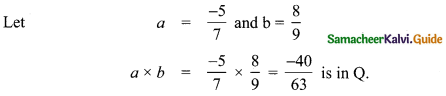∴ Closure property is true for rnultiplìcation of rational numbers.Question 2.
Verify the commutative property for addition and multiplication for the rational numbers $$\frac{-10}{11}$$ and $$\frac{-8{33}$$.
Let a = $$\frac{-10}{11}$$ and $$\frac{-8{33}$$ be the given rational numbers.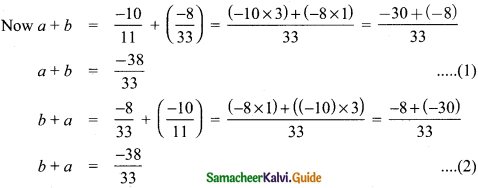From (1) and (2)
a + b = b + a and hence additionis commutative for rational numbers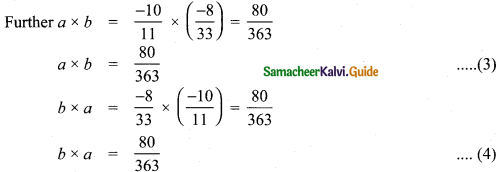From (3) and (4) a × b = b × a
Hence multiplication is commutative for rational numbers.Question 3.
Verify the associative property for addition and multiplication for the rational numbers $$\frac{-7}{9}, \frac{5}{6}$$ and $$\frac{-4}{3}$$.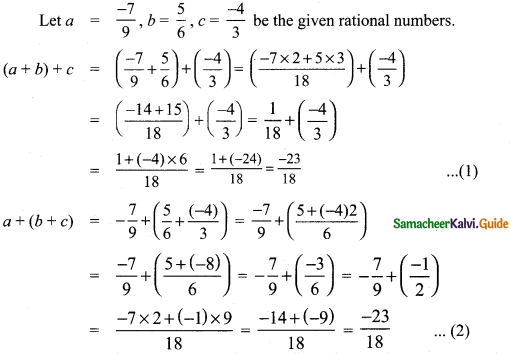From (1) and (2), (a + b) + c = a + (b + c) is true for rational numbers.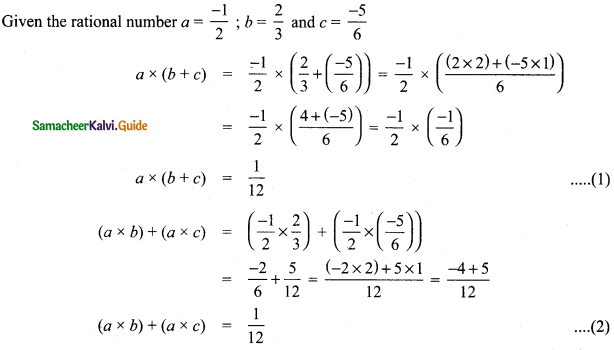From (1) and (2) (a × b) × c = (a × b) × c is true for rational numbers.
Thus associative property.Question 4.
Verify the distributive property a × (b + c) = (a × b) + (a + c) for the rational numbers a = $$\frac{-1}{2}$$, b = $$\frac{2}{3}$$ and c = $$\frac{-5}{6}$$.From (1) and (2) we have a × (b + c) = (a × b) + (a × c) is true
Hence multiplication is distributive over addition for rational numbers Q.Question 5.
Verify the identity property for addition and multiplication for the rational numbers $$\frac{15}{19}$$ and $$\frac{-18}{25}$$.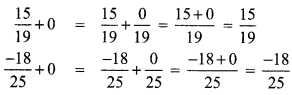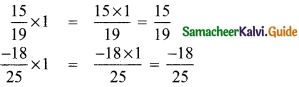Identify property for multiplication verified.

Question 6.
Verify the additive and multiplicative inverse property for the rational numbers $$\frac{-7}{17}$$ and $$\frac{17}{27}$$.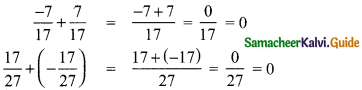Additive inverse for rational numbers verified.Mulplicative inverse for rational numbers verified.Objective Type Questions

Question 7.
Closure property is not true for division of rational numbers because of the number
(A) 1
(B) 1
(C) 0
(D) $$\frac { 1 }{ 2 }$$
(C) 0

Question 8.
$$\frac{1}{2}-\left(\frac{3}{4}-\frac{5}{6}\right) \neq\left(\frac{1}{2}-\frac{3}{4}\right)-\frac{5}{6}$$ illustrates that subtraction does not satisfy the ________ property for rational numbers.
(A) commutative
(B) closure
(C) distributive
(D) associative
(D) associativeQuestion 9.
Which of the following illustrates the inverse property for addition?
(A) $$\frac{1}{8}-\frac{1}{8}=0$$
(B) $$\frac{1}{8}+\frac{1}{8}=\frac{1}{4}$$
(C) $$\frac{1}{8}+0=\frac{1}{8}$$
(D) $$\frac{1}{8}-0=\frac{1}{8}$$
(A) $$\frac{1}{8}-\frac{1}{8}=0$$$$\frac{3}{4} \times\left(\frac{1}{2}-\frac{1}{4}\right)=\frac{3}{4} \times \frac{1}{2}-\frac{3}{4} \times \frac{1}{4}$$ illustrates that multiplication is distributive over